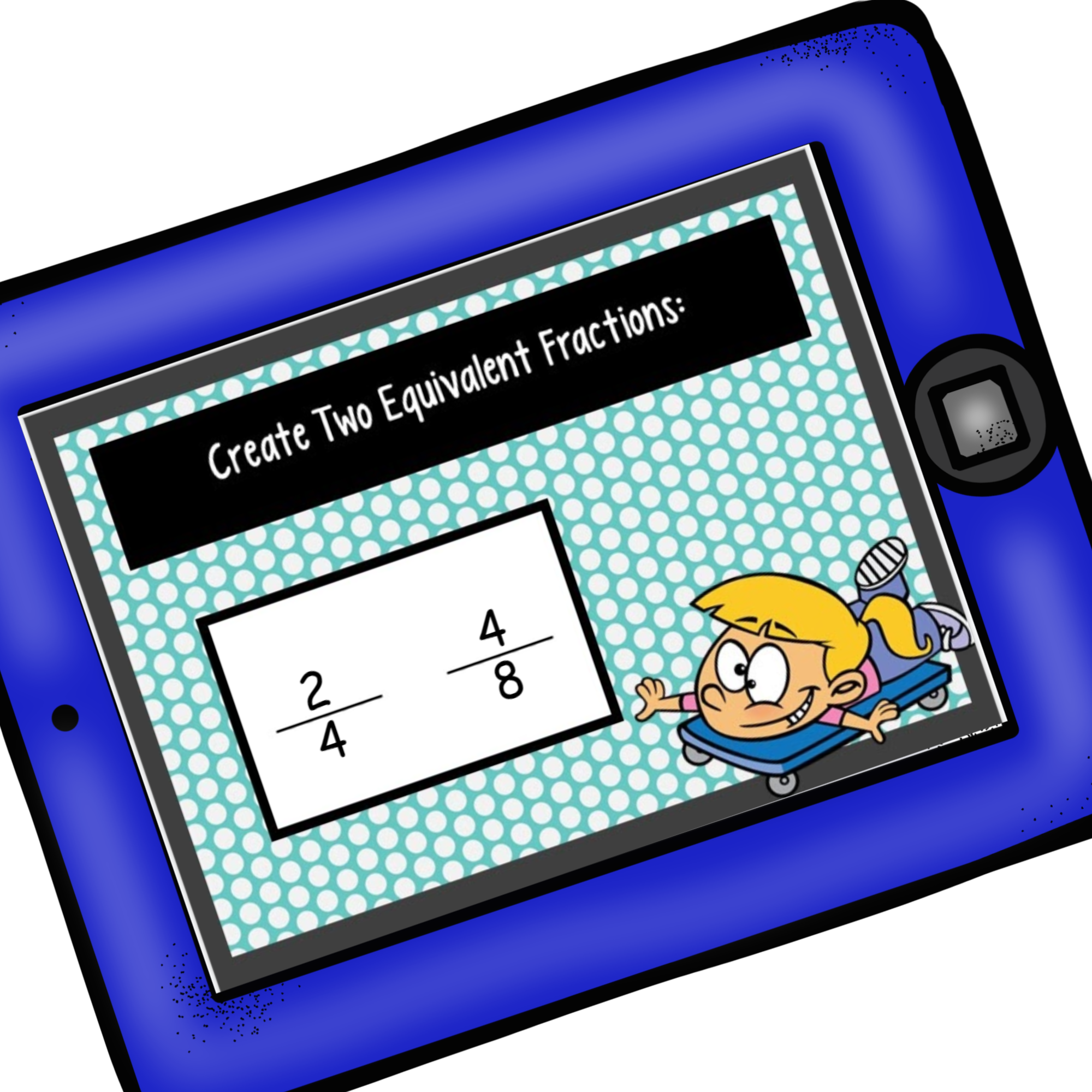# Fraction of the Day | Free Printable | Grades 3 – 5

45 Shares

This post has affiliate links.

## Fraction of the Day

Build fraction knowledge with your students or children by using Fraction of the Day. I walk you step-by-step through different fraction exercises you can have them do. I have provided free Google slides to use to practice fraction of the day exercises and also a free blank printable.

## Fraction Basics

He was sitting there, brow furrowed, and pencil tapping. We had been working on fractions for a few weeks, and I was sure he was going to be able to correctly fill the number line in.

Finally, after patiently or impatiently tongue-biting waiting, he started to scribble in his answers: 1/2, 1/3, 1/4, 1/5, 1/6….and on and one he wrote.

My heart sank.

He didn’t have a clue about fractions.

He didn’t have the basic understanding that the denominator tells us how many equal parts it takes to make a whole. We needed to go back to square one, and start building fraction knowledge.

Many children, even in upper elementary, have little or no understanding of fractions. They need to have repeated exposure to the basics every day.

The last time we met, we talked about giving the calendar a try in upper grades for a month. This calendar time is a great time to practice fraction basics, and I decided to throw in one more freebie to help you get started.

## Fraction of the Day Template

1. To get started, print off enough of the FREE printables for your students and place them in sheet protectors.
2. If you choose to use Google Slides, make a copy and then share it with your students. (I’m sorry, the Google Slides are not editable. Everything is a picture, except for the boxes the students type in. This way they can not move objects or the text around.)

## What Grades will Benefit from the Fraction of the Day?

The sheet is very open-ended and can be used for third, fourth, and fifth-grade students. It can be tailored to exactly what you are learning with fractions, and it helps build number sense in regard to fractions.

## How To Use Fraction of the Day Printable

Each morning, choose a fraction that the students will work on. You can create a fraction equation, ask them to create a model, create equivalent fractions, or work on fractions on a number line.

## Fraction Equation

You can make this as easy or as difficult as you like. You could leave it open-ended, or add some requirements. It could be as simple as using addition, or you could put in a fraction.

For example, if your fraction of the day is 1/2 you could tell your students that one equation must have 1/4. Think about the understanding of fractions that would be needed to do that.

(As a side note, when you go over the sheet each day, encourage students to not only share their equations but explain how they figured it out. There is a wealth of knowledge you can gain by hearing their thinking. It also may help struggling ones to hear their classmates think aloud.)

## Model for Fractions

Next, the kiddos get to draw a model. If you are using Google Slides, you may need to teach them how to insert a table, and use the fill button to color in certain boxes.

This step, just gets children to see the fraction as something other than numbers. Of course, 1/2 is quite easy. But 12/15 may not be…. and you could encourage them to find different ways to model 12/15 (equivalent fractions).

## Equivalent Fractions

Of course, we can’t work on fractions without having our kiddos find other equivalent fractions. So there is a slide or box that has our children finding TWO equivalent fractions.

And if you want to add a challenge….give them a fraction that needs to be simplified and require one of the equivalent fractions to be simplified.

(You can get more activities for equivalent fractions here.

## Fractions on a Number Line

Lastly, they will place their fraction on the number line. I would require that the fraction of the day not be the starting point. This requires the students to show fractions that come before and after.

It also reinforces that the denominator is the number of equal parts that make up the whole. You should see this on their number line.

If the fraction of the day is 3/4 then your students should be able to label the number line with 1/4, 2/4 or 1/2, 3/4, and then 1. I would not allow 4/4 as I want my little ones to demonstrate the understanding that 4/4 is one whole!

I’ve seen precious little ones (or sometimes they are bigger than me) write things like 1/2, 1/3, and then 1/4 on the number line. This is a red flag to me that we need to do some major backtracking.

There is something about fractions that this student does not understand, and our job is to figure it out.

If your students are getting it, add a challenge. Have the fraction of the day be 1/8 and do not allow them to start with it. See what they come up with. A negative number, 1/16, or another unique strategy that shows some creative thinking.

Get More Number Line Fraction Activities Here.

Fractions can be difficult, but with this type of repeated exposure found in this daily fraction printable, you will be surprised at the growth of knowledge in your kiddos. I can’t wait to hear how it goes for you.

## Fraction of the Day Google Slides

Below are the Google slides for the Fraction of the Day worksheets.

Fraction-of-the-Day-Goggle-Slides_Printable

## Fraction of the Day Printable

Below is the Fraction of the Day worksheet blank pages. You can print off the worksheets and your students can work on the fraction problem of the day.

Fraction-Of-The-Day45 Shares